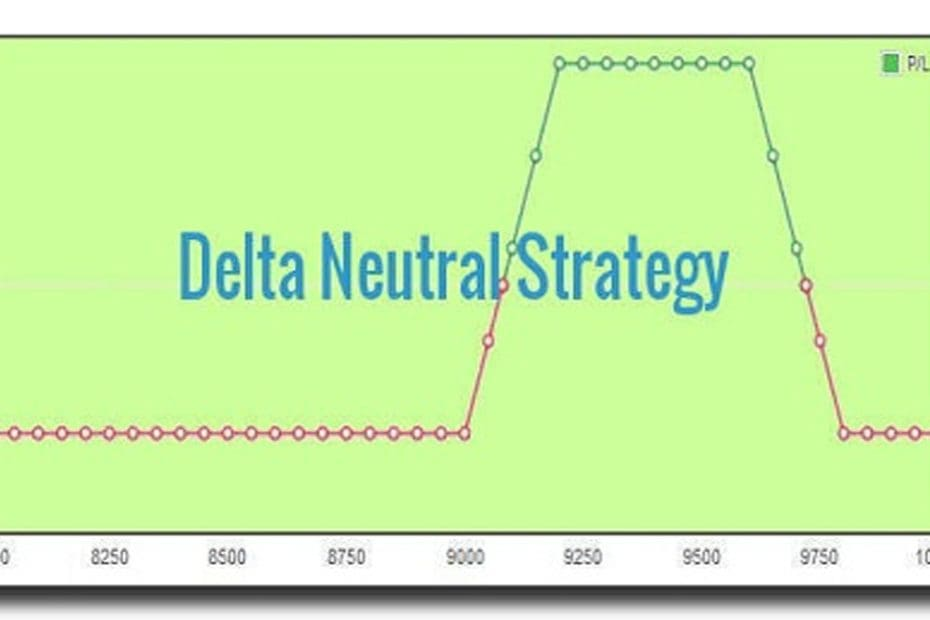Home » Blog » Trading » Trading Strategy » Delta Neutral Strategy – A Low Risk Hedged Strategy

# Delta Neutral Strategy – A Low Risk Hedged StrategyTaking a cue from my previous post on option greeks I start today with a strategy on how to use delta value to make delta neutral strategy. Such strategies may be standard options strategies or synthetic strategies. Probably the most popular option greek is Delta. Lion’s share of all option strategies uses Delta as the basis for the formulation of strategies.

Before moving further let us understand the significance of Delta. It measures the amount by which an option price changes for a unit price change of the underlying. To simplify we can say that for a call option of delta 0.25, it will increase by Rs 2.5 if the underlying stock price moves by Rs 10 or vice-versa. That call price will decrease by Rs 2.5/ put price will increase by Rs 2.5 or thereabouts for a Rs 10 decrease in price of underlying.

## Delta Neutral Strategy

Delta values of calls and puts of different strike prices are different and that holds true for all contracts of stocks and indices. For ATM strikes if we add absolute values of the delta of call and put, the total will be approximately 1. But delta values of calls are taken as positive whereas the put delta values are considered negative. So, if we add similar delta values (like ATM straddle), the total becomes nearly zero. Say, for example, Nifty CMP 9657, 9600 put has a delta value of 0.39 and Nifty 9700 call has a delta value of -0.43. Now if we take short strangle strategy, we short both 9600 put and 9700 call assuming that Nifty will be range bound and these two levels will not be breached in this expiry. If we add 0.39 – 0.43, we get -0.04 as the combined delta value. Hence the combined delta value near zero shows a delta neutral strategy. If Nifty expires within this range, we can pocket the credit we received by taking a short position at those strike prices. You can check deltas and create option strategies using Options Oracle Pasi.

Thus such a delta neutral strategy gives us the freedom to stick to our strategy even if Nifty moves + 100 points either way. The strategy holds true irrespective of market direction over a specified range. Calculated hedging increases risk-free zone.

We can use a delta neutral strategy to offset the effect of unpredictable variables like volatility, time decay from stock price or future. Say we buy 1 lot of SBI future @ 286 and simultaneously short 2 lots of 285 calls. The future has a delta of 1 and shorting 2 lots ATM call will give combined delta of approximately -1. Thus the combined strategy is delta neutral (+1-1=0) and lets you take a delta neutral stance to offset time decay. In addition through dynamic delta neutral strategy like gamma scalping, we offset volatility. There are numerous delta-neutral strategies. A professional trader uses such strategies to the highest effect.

For more information on delta neutral strategy visit here. For other trading strategies kindly visit previous posts.